# Cube

Calculate the surface cube with edge 11 dm.

Result

S =  726 dm2

#### Solution:Leave us a comment of example and its solution (i.e. if it is still somewhat unclear...):

Showing 0 comments:Be the first to comment!## Next similar examples:

1. The cube - simpleCalculate the surface of the cube measuring 15 centimeters.
2. Cube surface areaWall of cube has content area 99 cm square. What is the surface of the cube?
3. Cuboid box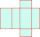Cuboid box have dimensions of 30 cm, 25 cm and 40 cm. Sketch its network and compute surface area of box.
4. Flood waterFlood waters in some US village meant that the homes had to evacuate 364 people. 50 of them stayed at elementary schools, 59 them slept with their friends and others went to relatives. How many people have gone to relatives?
5. Foot in busIt was 102 people on the bus. 28 girls had two dogs. A 11 girls had one dog. At the next stop seceded 5 dogs (even with their owners). They got two boys together with three dogs. The bus drove one driver. How many foot were in bus?
6. Line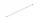How many parts of line divide 5 (different) points that lie on it?
7. Plane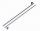On how many parts divide plane 6 parallels?
8. Math classificationIn 3A class are 27 students. One-third got a B in math and the rest got A. How many students received a B in math?
9. The resultHow many times I decrease the number 1632 to get the result 24?
10. Expressions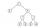Find out value of expressions if a = -1, b =2: x=b - 2a - ab y=a3 - b2 - 2ab z=a2 b3 - a3 b2 w=a + b + a3 - b2
11. Valid numberRound the 453874528 on 2 significant numbers.
12. Addition of Roman numbersAdded together and write as decimal number: LXVII + MLXIV
13. Ten pupils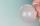10 pupils went to the store. 6 pupils bought lollipops and 9 pupils bought chewing gum. How many pupils have bought both lollipops and chewing gums (if everyone bought something)?
14. Circles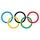For the circle c1(S1; r1=146 cm) and c2(S2; r2 = 144 cm) is distance of centers |S1S2| = 295 cm. Determine the distance between the circles.
15. CakesOn the bowl were a few cakes. Jane ate one-third of them, Dana ate a quarter of those cakes that remained. a) What part (of the original number of cakes) Dana ate? b) At least how many cakes could be (initially) on thebowl?
16. One frame5 picture frames cost € 12 more than three frames. How much cost one frame?
17. Eq1Solve equation: 4(a-3)=3(2a-5)